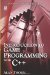# 1.1 Number Systems

## 1.1 Number Systems

Mathematics is often explained in school as the language of numbers, and while true, this description offers only a limited insight as to what mathematics really is. Mathematics, as we shall see, covers a broad area of subjects, including counting, measuring distances, and describing shapes.

One of the first historical uses of math was for counting; to determine how much of something existed, e.g., there are five people, or 10 apples, etc. Later, this system was extended to measure distances so people could understand spatial relationships. However, to express the concept of quantity, a system of numerals, or numbers, was required. The Romans used Roman numerals, the Greeks used letters and other symbols, but none of these systems survived antiquity completely. The numerals we use today find their origins in the Arabic numerals, such as 1, 2, 3, etc. These standard numerals as we know them today take two forms: cardinal and ordinal.

The cardinal numbers are used to answers questions such as "How many?" and are:

• 1, 2, 3, 4, 5, 6, 7, and so on.

The ordinal numbers are used to answer questions such as "Which one?" and are:

• 1st, 2nd, 3rd, 4th, 5th, 6th, 7th, and so on.

### 1.1.1 Decimal System (Base 10)

The standard numerals, also known as the counting numbers, are arranged in a system of notation. The system we use now is known as the decimal system, and every number in the decimal system can be composed from the following 10 numerals: 0, 1, 2, 3, 4, 5, 6, 7, 8, and 9. Whenever we write a number in decimal form we do so using a notation of place value. For example, the number 351 can be written in decimal as follows.

Hundreds

Tens

Units

3

5

1

Each column represents the next power of 10 from the one on the right (1 × 10, 10 × 10, 100 × 10), and this means smaller quantities are written on the right and larger ones on the left. 351 can be seen to represent 3 hundreds, 2 tens, and 1 unit.

Beyond hundreds, tens, and units, there are thousands, millions, billions, trillions, and so on. When a decimal number is greater than three digits, such as 4510 or 7900, it is typical to see a comma inserted every three digits from the right to break the number down and make it easier to read, such as 4,510 and 7,900. The comma system is extended to every three digits, as in 973,452,576,999.

 Note Each column, whether hundreds, tens, units, or whatever, is called a number's place value. If a number has 3 hundreds, no tens, and 2 units, it cannot be written as 32. It should appear as 302. The 0 is inserted into any column to indicate that there is no value.Introduction to Game Programming with C++ (Wordware Game Developers Library)
ISBN: 1598220322
EAN: 2147483647
Year: 2007
Pages: 225
Authors: Alan Thorn

Similar book on Amazon